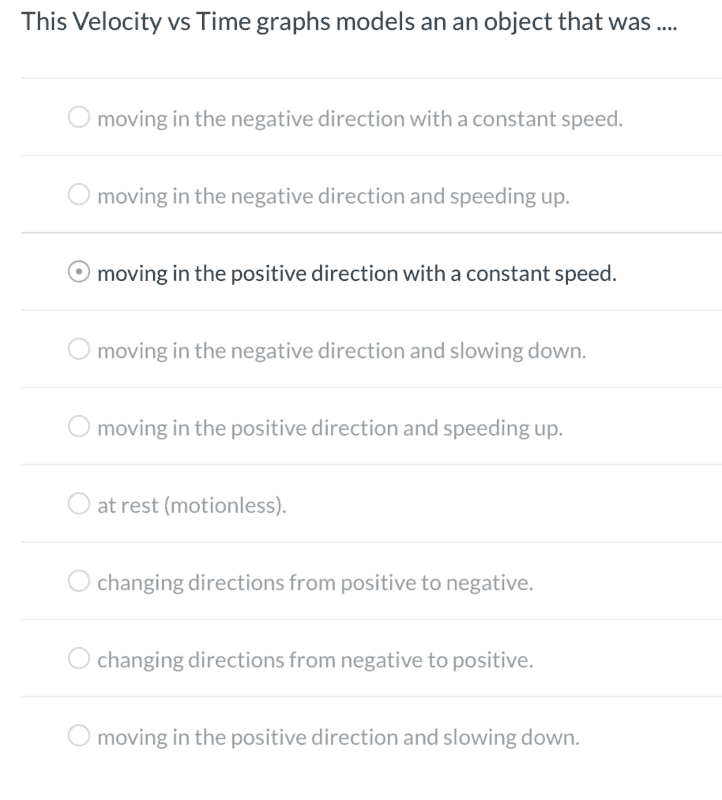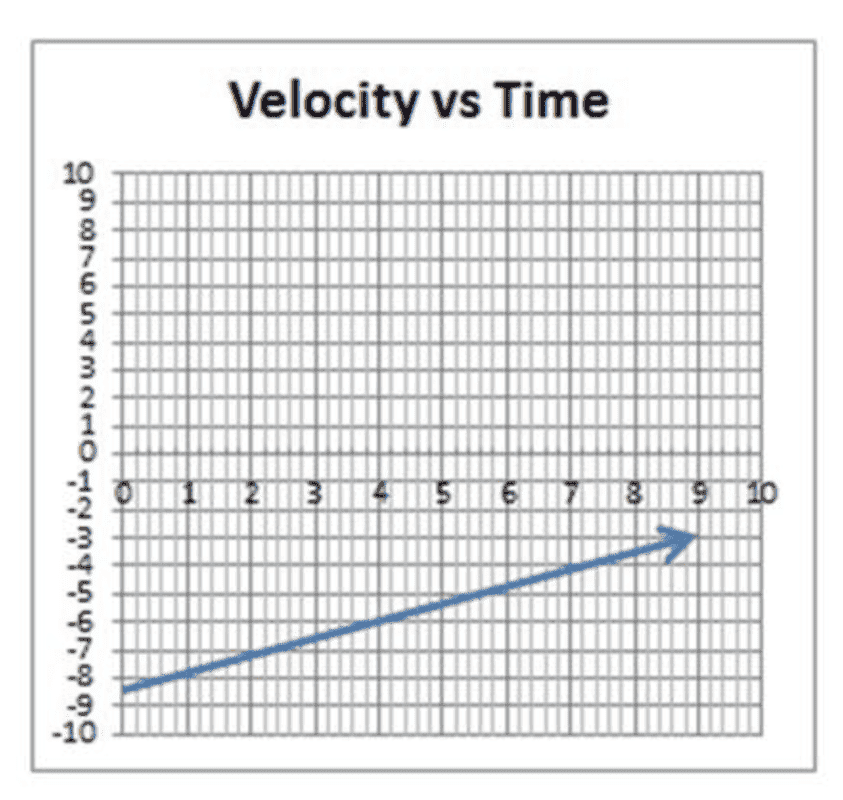# Velocity Vs Time Graphs

kalcorn
Homework Statement:
This Velocity vs Time graphs models an an object that was ....
Relevant Equations:
NoneThis is the graph I was given and the answer options. Since the graph is a straight line moving towards the positive direction I thought that meant it was traveling at a constant speed moving in the positive direction. That ended up being the wrong answer. Can anyone offer insight, I missed each question that was like this.

#### Attachments

Homework Helper
Gold Member
2022 Award
Constant speed means the speed isn't changing. If the speed is constant what is that constant value from the graph?

Homework Helper
Gold Member
graph is a straight line moving towards the positive direction
graph of what?
what is moving?
What does the graph represent?

Homework Helper
Gold Member
Homework Statement:: This Velocity vs Time graphs models an an object that was ...
Can anyone offer insight, I missed each question that was like this.
At time=0, the object had a velocity of -8.5 units: the negative sign suggests that the object was moving in a negative direction respect to some pre-set reference.

At time=9 units, the object had a velocity of -3.0 units: the negative sign suggests that the object was still moving in that same negative direction, but slowing down from the previous magnitude of velocity.

•PhysicsEnthusiast123 and Delta2
David Lewis
Since the graph is a straight line moving towards the positive direction I thought that meant it was traveling at a constant speed moving in the positive direction
On a position vs time graph, a straight line indicates constant speed.

•Delta2
Gold Member
I agree, the straight line (with both numbers increasing) indicates constant speed in a positive direction.
What's missing is labels, so I don't know if it represents a speed of 0.61 or 1.64
Is time vertical or horizontal in that graph? Doesn't say, which is fine because they don't ask.

Homework Helper
I agree, the straight line (with both numbers increasing) indicates constant speed in a positive direction.
On a position versus time graph, that would be correct. This is not a position versus time graph.

•Halc
Gold Member
On a position versus time graph, that would be correct. This is not a position versus time graph.
I stand corrected. The answer given by the OP is wrong, as was I. Both fooled by the same thing.
My comment about the lack of labeling is important now, as it gives different answers depending on which axis is the time and which is the velocity.

Gold Member
Apparently the student - as well as having to figure out the problem - has to figure out exactly what the problem is, in the first place. Seems to be a pande... umm, forget that.

Anyways...

Having a range from 10 to -10 would seem to indicate that - given the apparent simplicity of the problem - the vertical axis does not indicate Time.

Does that help ?

David Lewis
The vertical axis is velocity and the horizontal axis is time because the graph is titled Velocity vs Time. (The conventional ordering is "dependent variable" vs "independent variable.")

•Lnewqban